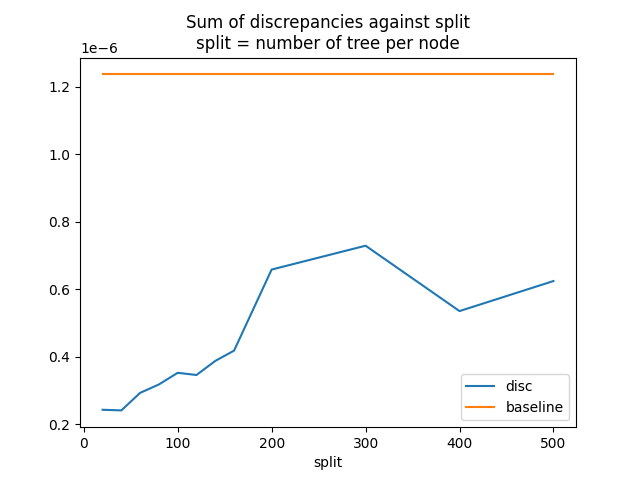# Convert a pipeline with a LightGBM regressor#

The discrepancies observed when using float and TreeEnsemble operator (see Issues when switching to float) explains why the converter for LGBMRegressor may introduce significant discrepancies even when it is used with float tensors.

Library lightgbm is implemented with double. A random forest regressor with multiple trees computes its prediction by adding the prediction of every tree. After being converting into ONNX, this summation becomes \left[\sum\right]_{i=1}^F float(T_i(x)), where F is the number of trees in the forest, T_i(x) the output of tree i and \left[\sum\right] a float addition. The discrepancy can be expressed as D(x) = |\left[\sum\right]_{i=1}^F float(T_i(x)) - \sum_{i=1}^F T_i(x)|. This grows with the number of trees in the forest.

To reduce the impact, an option was added to split the node TreeEnsembleRegressor into multiple ones and to do a summation with double this time. If we assume the node if split into a nodes, the discrepancies then become D'(x) = |\sum_{k=1}^a \left[\sum\right]_{i=1}^{F/a} float(T_{ak + i}(x)) - \sum_{i=1}^F T_i(x)|.

## Train a LGBMRegressor#

import packaging.version as pv
import warnings
import timeit
import numpy
from pandas import DataFrame
import matplotlib.pyplot as plt
from tqdm import tqdm
from lightgbm import LGBMRegressor
from onnxruntime import InferenceSession
from skl2onnx import to_onnx, update_registered_converter
from skl2onnx.common.shape_calculator import calculate_linear_regressor_output_shapes  # noqa
from onnxmltools import __version__ as oml_version
from onnxmltools.convert.lightgbm.operator_converters.LightGbm import convert_lightgbm  # noqa

N = 1000
X = numpy.random.randn(N, 20)
y = (numpy.random.randn(N) +
numpy.random.randn(N) * 100 * numpy.random.randint(0, 1, 1000))

reg = LGBMRegressor(n_estimators=1000)
reg.fit(X, y)

LGBMRegressor(n_estimators=1000)
In a Jupyter environment, please rerun this cell to show the HTML representation or trust the notebook.

## Register the converter for LGBMClassifier#

The converter is implemented in onnxmltools: onnxmltools…LightGbm.py. and the shape calculator: onnxmltools…Regressor.py.

def skl2onnx_convert_lightgbm(scope, operator, container):
options = scope.get_options(operator.raw_operator)
if 'split' in options:
if pv.Version(oml_version) < pv.Version('1.9.2'):
warnings.warn(
"Option split was released in version 1.9.2 but %s is "
"installed. It will be ignored." % oml_version)
operator.split = options['split']
else:
operator.split = None
convert_lightgbm(scope, operator, container)

update_registered_converter(
LGBMRegressor, 'LightGbmLGBMRegressor',
calculate_linear_regressor_output_shapes,
skl2onnx_convert_lightgbm,
options={'split': None})


## Convert#

We convert the same model following the two scenarios, one single TreeEnsembleRegressor node, or more. split parameter is the number of trees per node TreeEnsembleRegressor.

model_onnx = to_onnx(reg, X[:1].astype(numpy.float32),
target_opset={'': 14, 'ai.onnx.ml': 2})
model_onnx_split = to_onnx(reg, X[:1].astype(numpy.float32),
target_opset={'': 14, 'ai.onnx.ml': 2},
options={'split': 100})


## Discrepancies#

sess = InferenceSession(model_onnx.SerializeToString())
sess_split = InferenceSession(model_onnx_split.SerializeToString())

X32 = X.astype(numpy.float32)
expected = reg.predict(X32)
got = sess.run(None, {'X': X32}).ravel()
got_split = sess_split.run(None, {'X': X32}).ravel()

disp = numpy.abs(got - expected).sum()
disp_split = numpy.abs(got_split - expected).sum()

print("sum of discrepancies 1 node", disp)
print("sum of discrepancies split node",
disp_split, "ratio:", disp / disp_split)


Out:

sum of discrepancies 1 node 0.00011677806003327501
sum of discrepancies split node 4.402241789117978e-05 ratio: 2.6526952772549177


The sum of the discrepancies were reduced 4, 5 times. The maximum is much better too.

disc = numpy.abs(got - expected).max()
disc_split = numpy.abs(got_split - expected).max()

print("max discrepancies 1 node", disc)
print("max discrepancies split node", disc_split, "ratio:", disc / disc_split)


Out:

max discrepancies 1 node 1.0208151959290035e-06
max discrepancies split node 3.5547470744745624e-07 ratio: 2.8716957199546838


## Processing time#

The processing time is slower but not much.

print("processing time no split",
timeit.timeit(
lambda: sess.run(None, {'X': X32}), number=150))
print("processing time split",
timeit.timeit(
lambda: sess_split.run(None, {'X': X32}), number=150))


Out:

processing time no split 0.7822519999999997
processing time split 0.8093972000000065


## Split influence#

Let’s see how the sum of the discrepancies moves against the parameter split.

res = []
for i in tqdm(list(range(20, 170, 20)) + [200, 300, 400, 500]):
model_onnx_split = to_onnx(reg, X[:1].astype(numpy.float32),
target_opset={'': 14, 'ai.onnx.ml': 2},
options={'split': i})
sess_split = InferenceSession(model_onnx_split.SerializeToString())
got_split = sess_split.run(None, {'X': X32}).ravel()
disc_split = numpy.abs(got_split - expected).max()
res.append(dict(split=i, disc=disc_split))

df = DataFrame(res).set_index('split')
df["baseline"] = disc
print(df)


Out:

  0%|                                                           | 0/12 [00:00<?, ?it/s]
8%|####2                                              | 1/12 [00:02<00:24,  2.25s/it]
17%|########5                                          | 2/12 [00:04<00:22,  2.25s/it]
25%|############7                                      | 3/12 [00:06<00:19,  2.15s/it]
33%|#################                                  | 4/12 [00:08<00:17,  2.17s/it]
42%|#####################2                             | 5/12 [00:10<00:14,  2.11s/it]
50%|#########################5                         | 6/12 [00:12<00:12,  2.14s/it]
58%|#############################7                     | 7/12 [00:14<00:10,  2.09s/it]
67%|##################################                 | 8/12 [00:17<00:08,  2.10s/it]
75%|######################################2            | 9/12 [00:18<00:06,  2.05s/it]
83%|#########################################6        | 10/12 [00:21<00:04,  2.08s/it]
92%|#############################################8    | 11/12 [00:23<00:02,  2.04s/it]
100%|##################################################| 12/12 [00:25<00:00,  2.05s/it]
100%|##################################################| 12/12 [00:25<00:00,  2.10s/it]
disc  baseline
split
20     1.730885e-07  0.000001
40     1.790506e-07  0.000001
60     2.863737e-07  0.000001
80     2.863737e-07  0.000001
100    3.554747e-07  0.000001
120    4.524478e-07  0.000001
140    4.174692e-07  0.000001
160    3.860974e-07  0.000001
200    3.477843e-07  0.000001
300    5.396620e-07  0.000001
400    8.225882e-07  0.000001
500    6.640952e-07  0.000001


Graph.

ax = df.plot(title="Sum of discrepancies against split\n"
"split = number of tree per node")

# plt.show()Total running time of the script: ( 0 minutes 31.683 seconds)

Gallery generated by Sphinx-Gallery